To check if a point is inside a box in Unity, you will probably use `BoxCollder.Bounds.Contains`. Although, the results you get can be really confusing, like the following: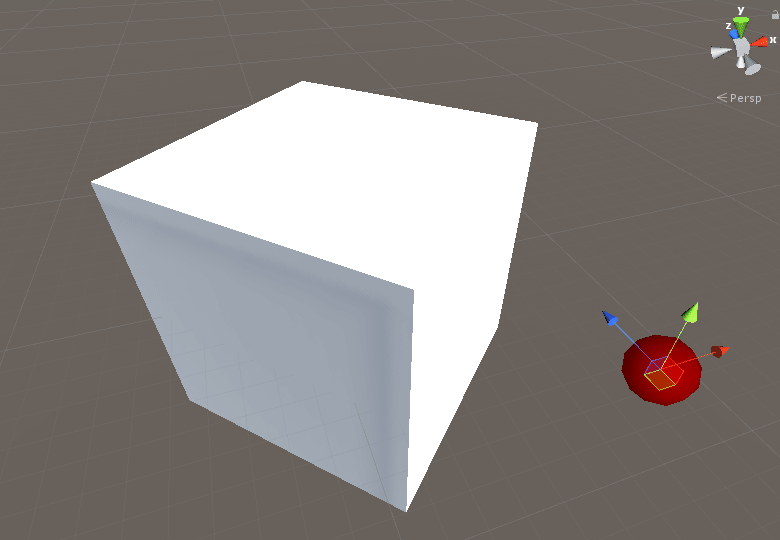The code running for the above gif is this one (attached to moving point):

``````[SerializeField] BoxCollider BoxCollider;

private void OnDrawGizmosSelected()
{
bool isPointInside = BoxCollider.bounds.Contains(transform.position);

Gizmos.color = isPointInside ? Color.green : Color.red;
Gizmos.DrawSphere(transform.position, 0.1f);
}
``````

I will try to explain here as clearly as possible what’s happening and how to fix it.

## What’s happening ?

First of all, you may have noticed that the results are wrong only when the box is rotated or scaled. Here is the same code running on a box with rotation `0, 0, 0`: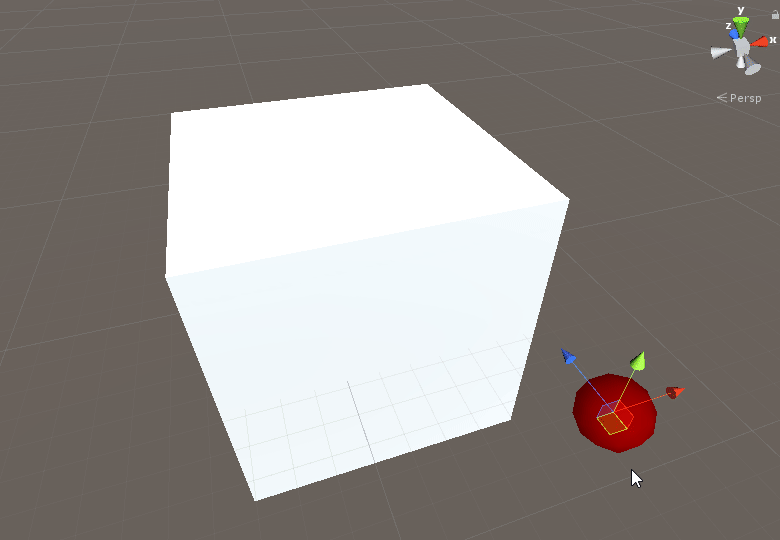The reason is that the bounds of the box collider is axis-aligned. This means that it can’t be rotated and so it won’t take into account your box rotation.

It can be really confusing, especially when you see this that green wireframe following the box closely when rotating it: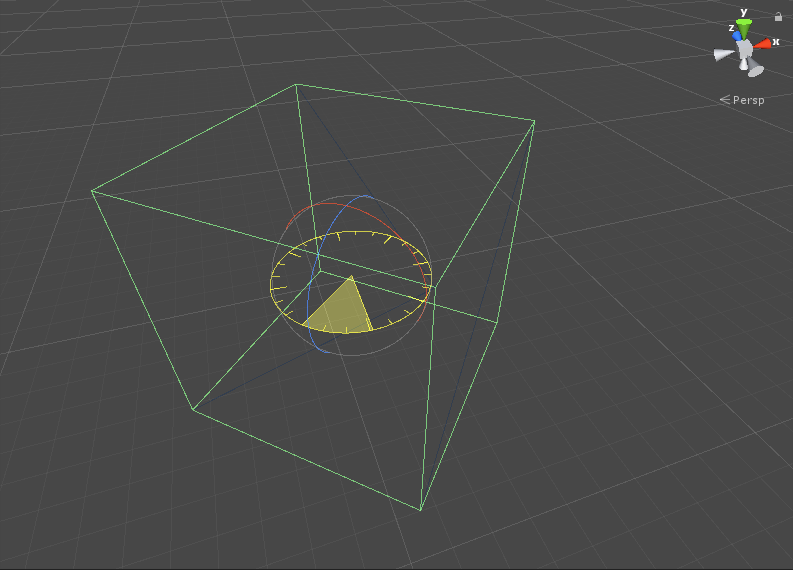Moreover, the bounds attached to the box collider are closely following the space occupied by the mesh. Which means that the bounds size is changing as the object is rotating ! That’s because bounds can’t be rotated, the only way it has to surround the mesh is to change its size. See how the bounds of the box really look like in blue: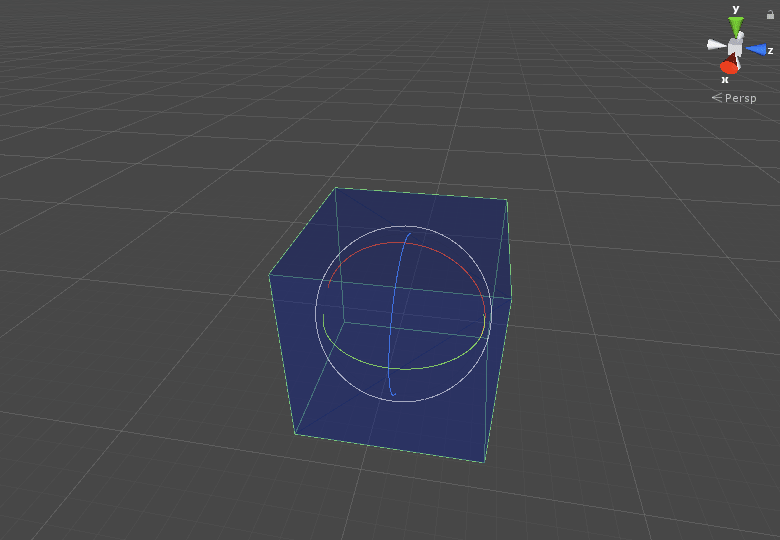The code used for the above gif is the following:

``````[SerializeField] BoxCollider BoxCollider;

private void OnDrawGizmosSelected()
{
Gizmos.color = new Color(0.1f, 0.2f, 0.8f, 0.5f);
Gizmos.DrawCube(BoxCollider.bounds.center, BoxCollider.bounds.size);
}
``````

## How to fix it ?

A way to fix it is to place the point in the box space. By doing so, the rotation is correctly taken into account and the results are correct. Although, it is a more expensive call as you have to transform the points. The bounds is also re-created to make sure the size is correct.

``````// Place the point in box space.
Vector3 pointBoxSpace = BoxCollider.transform.InverseTransformPoint(transform.position);

// Compute the real bounds, not incorrectly affected by the rotation.
Bounds correctBounds = new Bounds(BoxCollider.center, BoxCollider.size);

bool isPointInside = correctBounds.Contains(pointBoxSpace);
``````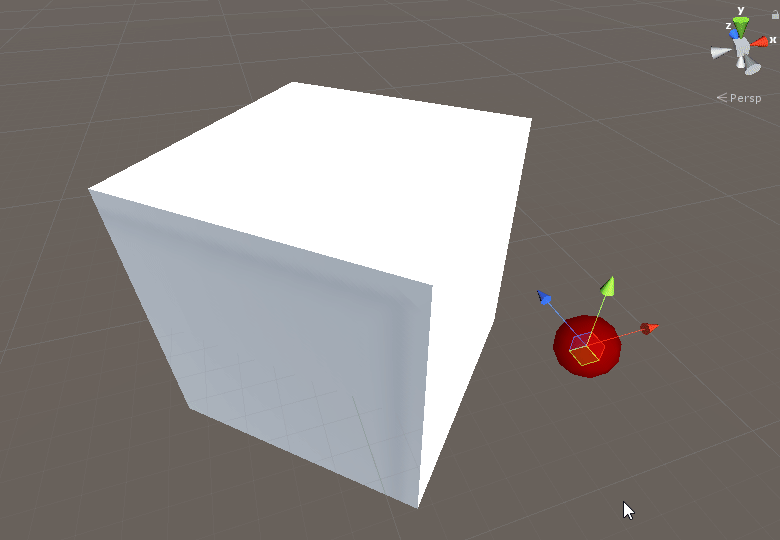## What about box intersection ?

Testing intersection between multiple boxes using `BoxCollider.Bounds.Intersect` is easy, as long as the boxes are in the same space. But if one of the boxes doesn’t have a uniform rotation (0, 90, 180, 270), then there is no easy and working way to test for intersection.

You would need to create a oriented box (OBB as opposed to AABB) from the box collider and then write an intersection test for these boxes. More info here in the An Oriented Bounding Box (OBB) Intersection Test section.